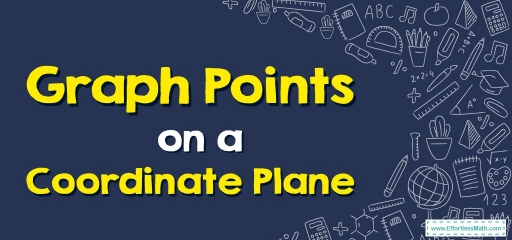# Graph Points on a Coordinate Plane## What’s a Coordinate Plane?

Coordinate planes are two-dimensional surfaces created using $$2$$ number lines. It’s created whenever a horizontal line known as the $$X$$-axis as well as a vertical line known as a $$Y$$-axis intersect at a point known as the origin.

The numerals on a coordinate grid get utilized to find points. You can utilize a coordinate plane to graph points, lines, etc. It behaves like a map and produces accurate directions from one place to another.

## What are Coordinates?

Coordinates are a collection of $$2$$ values that find a precise spot on a coordinate plane grid, more well-known as a coordinate plane.

Points in coordinate planes are named via their ordered pair $$(x, y)$$, written inside parentheses, equivalent to the $$X$$-coordinate along with the $$Y$$-coordinate.

The coordinates could be positive, negative, or zero, dependent on the position of the point in the respective quadrant.

## Quadrants on a Coordinate Plane

Quadrants can be described as an area/part of a cartesian or a coordinate plane achieved whenever the $$2$$ axes intersect with each other.

• $$1^{st}$$ quadrant: $$x > 0, y > 0$$
• $$2nd$$ quadrant: $$x < 0, y > 0$$
• $$3rd$$ quadrant: $$x < 0, y < 0$$
• $$4th$$ quadrant: $$x > 0, y < 0$$

## Finding a Point on a Coordinate Plane

Since we’re already acquainted with coordinate planes and their parts, now we can talk about the way to identify points on a coordinate plane. To find a point on a coordinate plane, follow the steps presented below:

• Step one: Find a point.
• Step two: Find a quadrant by looking at the signs of its $$X$$ and $$Y$$ coordinates.
• Step three: Find its $$X$$-coordinate or abscissa of the point via reading the number of units the point is to the right/left of the origin along its $$X$$-axis.
• Step four: Find its $$Y$$-coordinate or the ordinate of the point via reading the number of units the point is below/above the origin along its $$Y$$-axis.

## Crucial Points on a Coordinate Plane:

• The $$1$$st quadrant $$(+, +)$$ called the positive coordinates quadrant is symbolized by the Roman numeral $$I$$.
• The $$2$$nd quadrant $$(-, +)$$ is characterized by the Roman numeral $$II$$.
• The $$3$$rd quadrant $$(-, -)$$ is characterized by the Roman numeral $$III$$.
• The $$4$$th quadrant $$(+, -)$$ is characterized by the Roman numeral $$IV$$.
• The coordinates of any point get put into brackets.

### Graph Points on a Coordinate Plane – Example:

Plot each point on the coordinate grid.

$$A (1,5)$$, $$B (3,3)$$, $$C(4,3)$$, $$D (5,2)$$

Solution:

First, we draw a coordinate axis so that the horizontal axis is the $$x$$-axis and the vertical axis is the $$y$$-axis. Each point with coordinates $$(x, y)$$ is defined on the axis so that $$x$$ denotes the number of units the point is to the right/left of the origin along its $$x$$-axis and $$y$$ denotes the number of units the point is below/above the origin along its $$y$$-axis. For example, to specify a point $$(1, 5)$$ from the origin of the coordinates, we must move one unit to the right and five units to the top.

## Exercises for Graph Points on a Coordinate Plane

Plot each point on the coordinate grid.

1. $$\color{blue}{A(5,3), B(1,8), C(7,2)}$$
2. $$\color{blue}{A(7,4), B(6,1), C(4,2)}$$

1)

2)

### What people say about "Graph Points on a Coordinate Plane - Effortless Math: We Help Students Learn to LOVE Mathematics"?

No one replied yet.

X
30% OFF

Limited time only!

Save Over 30%

SAVE $5 It was$16.99 now it is \$11.99